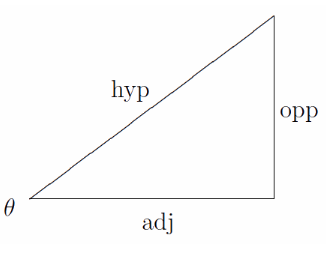# Sin Theta Formula

As per the sin theta formula, sin of an angle θ, in a right-angled triangle is equal to the ratio of opposite side and hypotenuse. The sine function is one of the important trigonometric functions apart from cos and tan. Here we will discuss finding sine of any angle, provided the length of the sides of the right triangle.

## Sin x Formula:

It is one of the formulae of the Sin function from the six other trigonometric functions.

 Sin $$\begin{array}{l}\Theta\end{array}$$ = $$\begin{array}{l}\frac{Opposite Side}{Hypotenuse}\end{array}$$## Sin angle Questions

Example: If cos x = 4/5, Find the value of Sin x?

Solution: Using Trigonometric identities: Sin2x = 1- Cos2x

Sin2x = 1 – (4/5)2

= 1 – 16/25

= (25 – 16) / 25

= 9/25

Sin x =

$$\begin{array}{l}\sqrt{9/25}\end{array}$$

= 3/5

Example 2: If Cosec x = 4/7, find the Sin x?

Solution: As Cosec x = 1/sin x

= 1/ 4/7

= 7/4

To Explore other trigonometric functions and its formulas, visit BYJU’S.